# Please explain the correct answer for both questions. Thank you! Incorrect Question 7 0/1 pts The...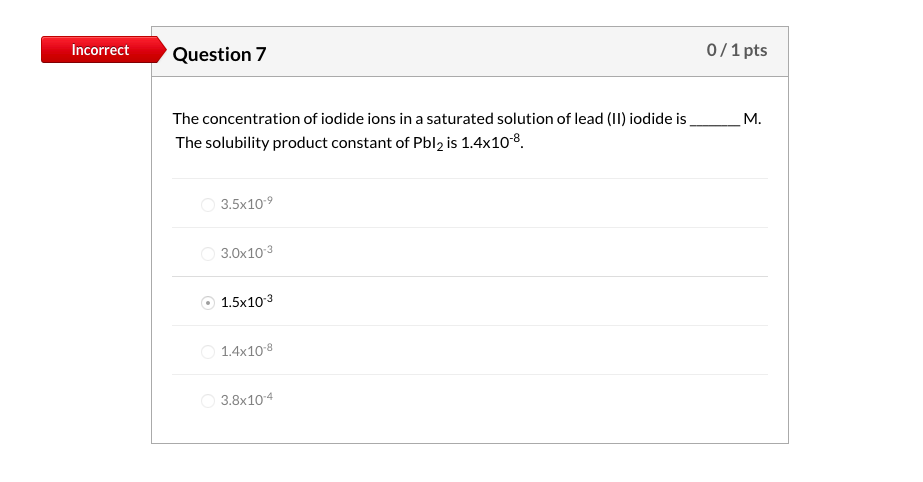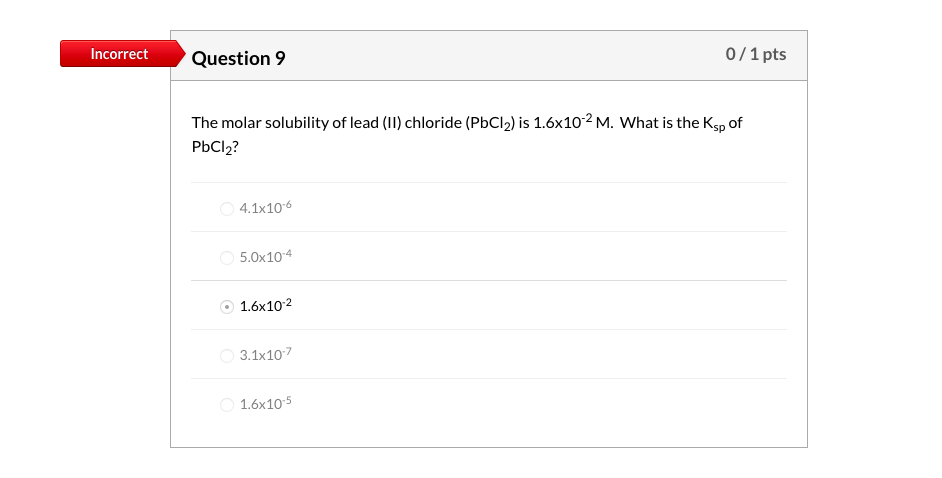Please explain the correct answer for both questions. Thank you!

Incorrect Question 7 0/1 pts The concentration of iodide ions in a saturated solution of lead (11) iodide is_ The solubility product constant of Pblz is 1.4x10-8. 3.5x10-9 3.0x10-3 1.5x103 1.4x10-8 3.8x10-4
Incorrect Question 9 0/1 pts The molar solubility of lead () chloride (PbCl2) is 1.6x102 M. What is the Ksp of PbCl2? 4.1x10-6 5.0x10-4 1.6x10-2 3.1x107 1.6x10-5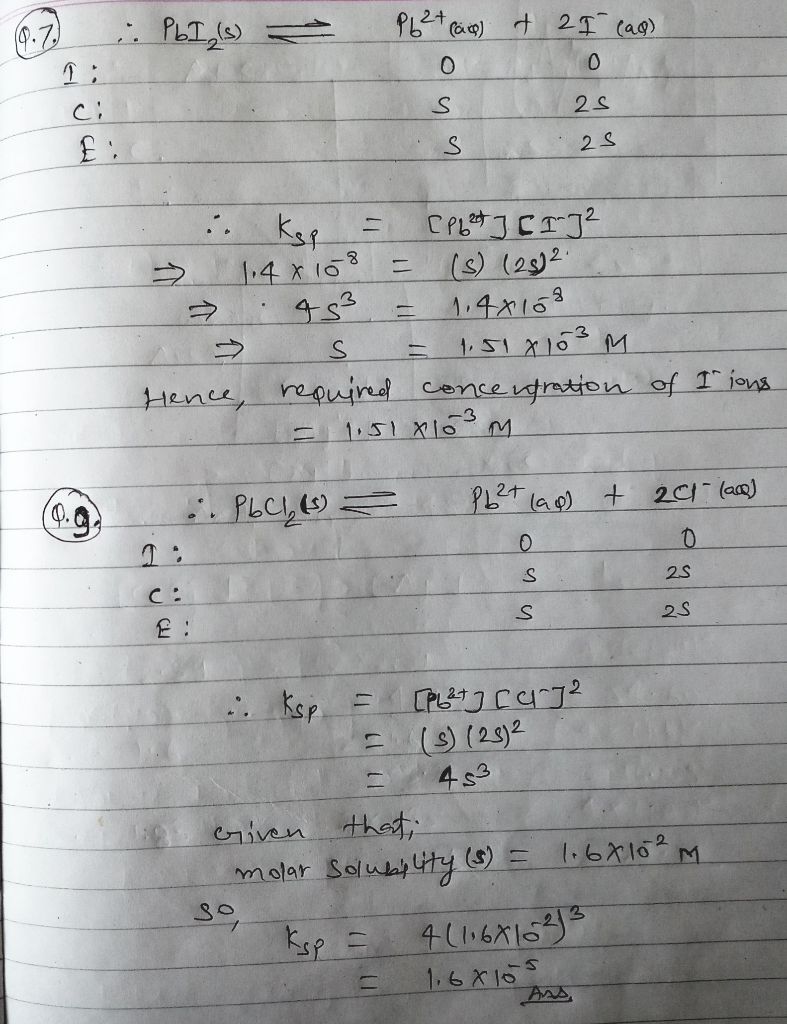#### Earn Coin

Coins can be redeemed for fabulous gifts.

Similar Homework Help Questions
• ### Please explain the correct answer. Thank you! Incorrect Question 2 0/1 pts What change will be...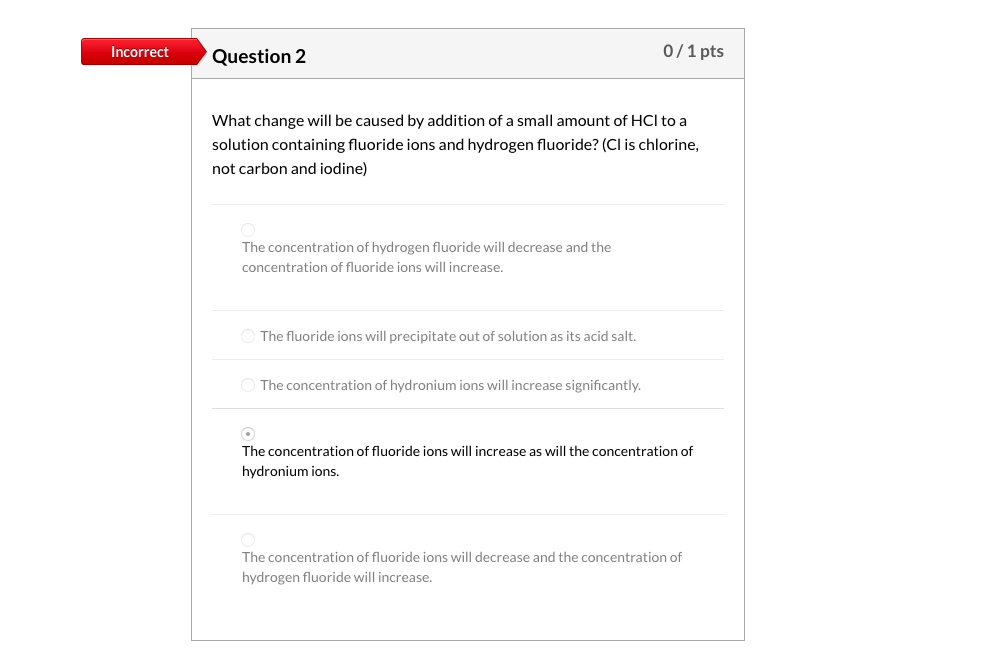Please explain the correct answer. Thank you! Incorrect Question 2 0/1 pts What change will be caused by addition of a small amount of HCl to a solution containing fluoride ions and hydrogen fluoride? (Cl is chlorine, not carbon and iodine) The concentration of hydrogen fluoride will decrease and the concentration of fluoride ions will increase. The fluoride ions will precipitate out of solution as its acid salt. The concentration of hydronium ions will increase significantly. The concentration of fluoride...

• ### Chapter 15 Question 9 1)A saturated solution of lead(II) chloride, PbCl2, was prepared by dissolving solid PbCl2 in wa...

Chapter 15 Question 9 1)A saturated solution of lead(II) chloride, PbCl2, was prepared by dissolving solid PbCl2 in water. The concentration of Pb2+ ion in the solution was found to be 1.62×10−2 M . Calculate Ksp for PbCl2. 2)The value of Ksp for silver sulfate, Ag2SO4, is 1.20×10−5. Calculate the solubility of Ag2SO4 in grams per liter.

• ### Please explain the correct answer to both questions. Thank you! Incorrect Question 19 0/1 pts The...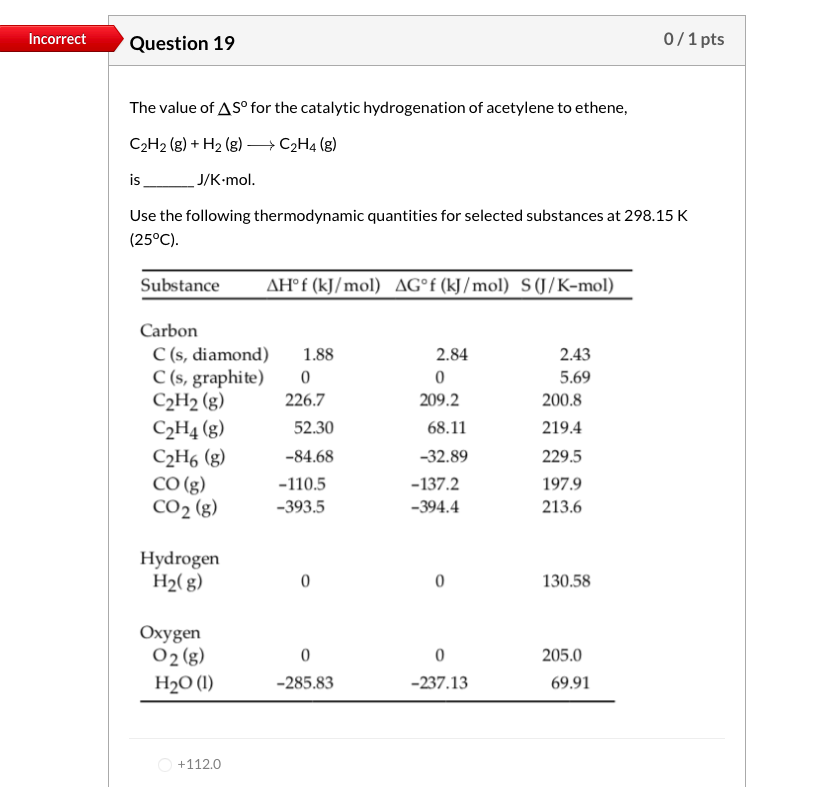Please explain the correct answer to both questions. Thank you! Incorrect Question 19 0/1 pts The value of A Sº for the catalytic hydrogenation of acetylene to ethene, C2H2(g) + H2(g) →C2H4 (g) is_ J/K.mol. Use the following thermodynamic quantities for selected substances at 298.15 K (25°C). Substance AHºf (kJ/mol) AG°f (kJ/mol) S (J/K-mol) 2.84 Carbon C(s, diamond) 1.88 C(s, graphite) 0 C2H2(g) 226.7 C2H4 (g) 52.30 C2H6 (g) -84.68 CO(g) -110.5 CO2 (g) -393.5 209.2 68.11 -32.89 -137.2 -394.4...

• ### Please explain the correct answer for both questions. Thank you! Incorrect Question 5 0/1 pts A...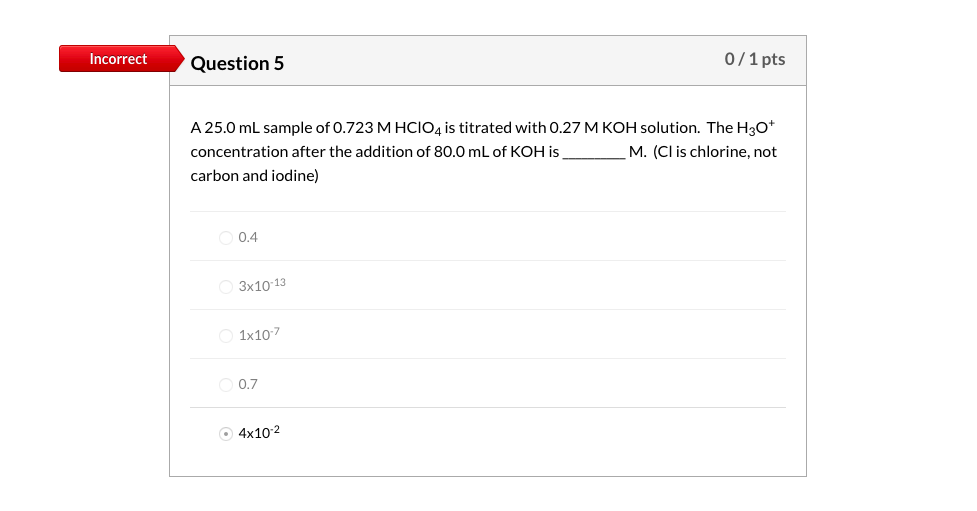Please explain the correct answer for both questions. Thank you! Incorrect Question 5 0/1 pts A 25.0 mL sample of 0.723 M HCIO4 is titrated with 0.27 M KOH solution. The H30* concentration after the addition of 80.0 mL of KOH is M. (Cl is chlorine, not carbon and iodine) 0.4 3x10-13 1x107 0.7 4x10-2 Incorrect Question 6 0/1 pts What could be the pKof this weak acid according to the titration curve below? Weak Acid Titration Curve PH 10...

• ### 1) Use the References to access important values if needed for this question. The maximum amount...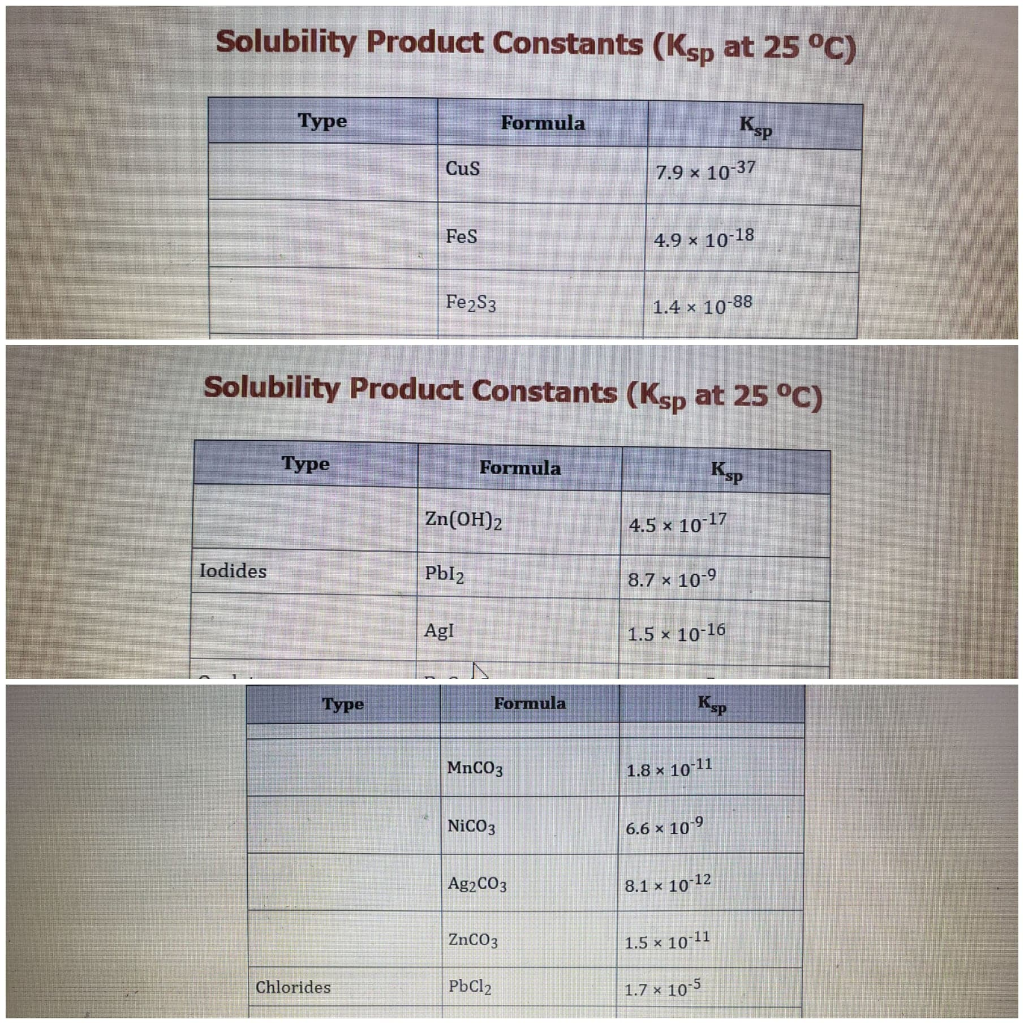1) Use the References to access important values if needed for this question. The maximum amount of lead chloride that will dissolve in a 0.138 M ammonium chloride solution is M. 2) Use the References to access important values if needed for this question. The molar solubility of lead iodide in a 0.138 M ammonium iodide solution is M. 3) Use the References to access important values if needed for this question. The molar solubility of iron(III) sulfide in a...

• ### #5 Write the solubility product expression for PbCl2. Using the concentration for the Pb+2 and Cl-...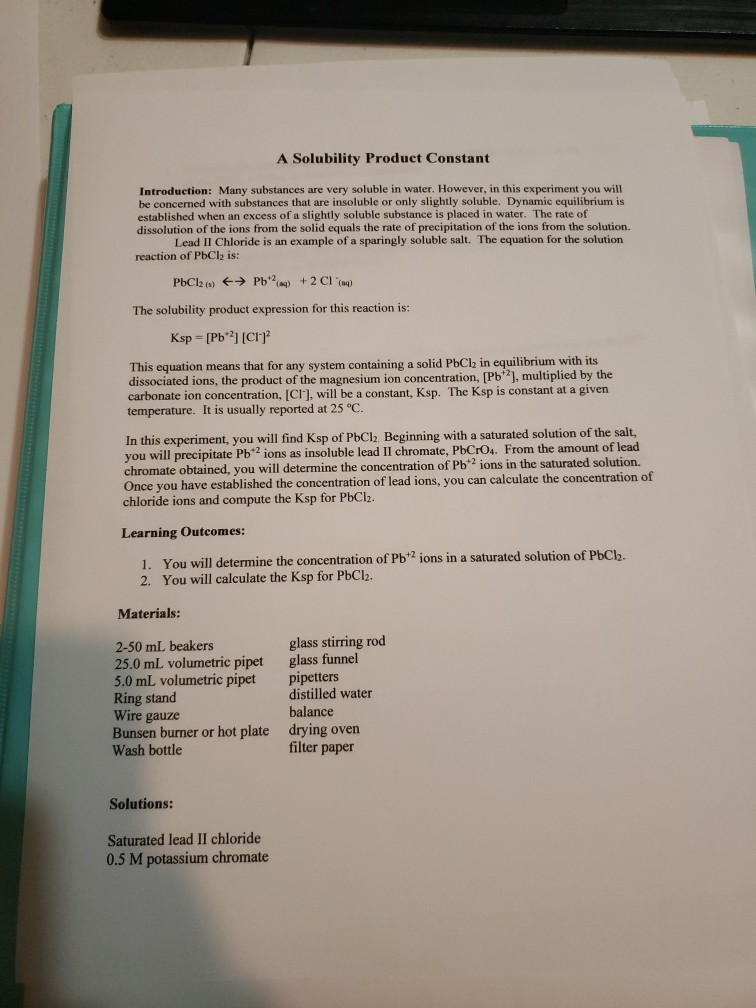#5 Write the solubility product expression for PbCl2. Using the concentration for the Pb+2 and Cl- ions, solve for your experimental Ksp. #6 Using your book, find the theoretical Ksp for PbCl2 to determine your percent error A Solubility Product Constant Introduction: Many substances are very soluble in water. However, in this experiment you will be concerned with substances that are insoluble or only slightly soluble. Dynamic equilibrium is established when an excess of a slightly soluble substance is placed...

• ### Please explain the correct answer to all three questions. Thank you! Incorrect Question 11 0/1 pts...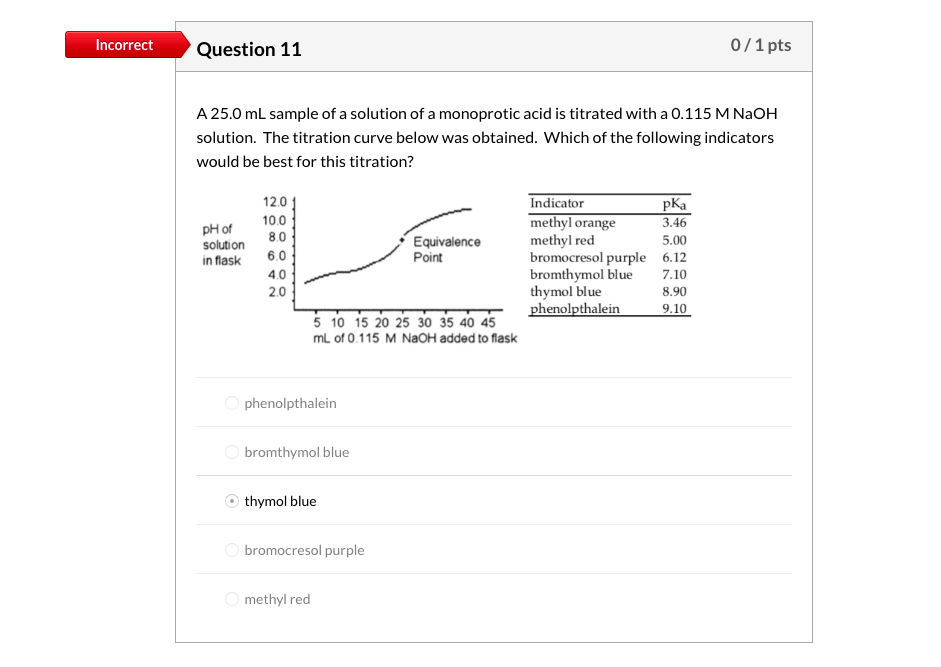Please explain the correct answer to all three questions. Thank you! Incorrect Question 11 0/1 pts A 25.0 mL sample of a solution of a monoprotic acid is titrated with a 0.115 M NaOH solution. The titration curve below was obtained. Which of the following indicators would be best for this titration? 12.01 10.0 8.0 pH of solution in flask 6.01 Equivalence Point Indicator methyl orange methyl red bromocresol purple bromthymol blue thymol blue phenolpthalein pka 3.46 5.00 6.12 7.10...

• ### Question 9 3 pts HOCl(aq) +H*(aq) + OCI (aq) The ionization of hypochlorous acid represented above...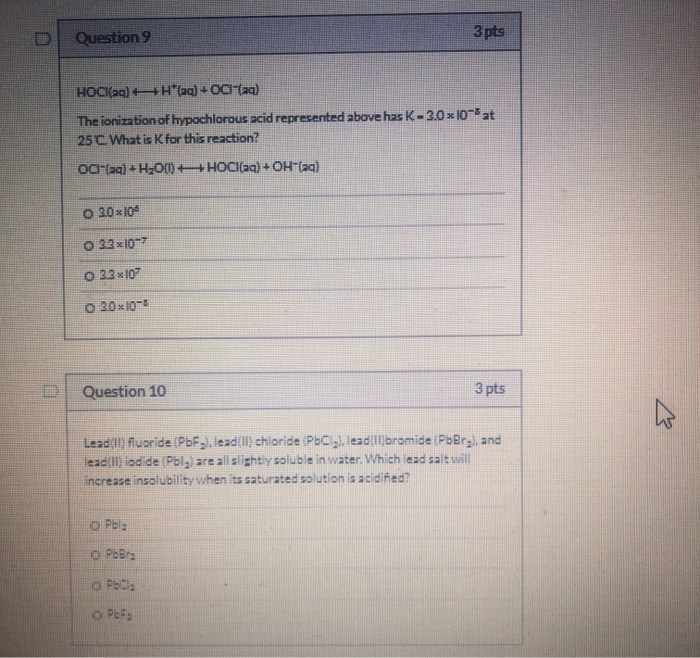Question 9 3 pts HOCl(aq) +H*(aq) + OCI (aq) The ionization of hypochlorous acid represented above has K - 3.0x10-8 at 25 C. What is K for this reaction? OC-(aq) +H300) +HOCl(aq) + OH-(aq) O 3.0x100 0 33x10-> O 33x10 0 3.0x10-3 Question 10 3 pts W Lead() fluoride (PbF), lead(II) chloride ( PC), lead()bromide (PbBrg, and lead(ll) iodide (Pbly) are all slightly soluble in water. Which lead salt will increase insolubility when its saturated solution is acidified? ОРЫla O...

• ### Question 9 3 pts HOCl(aq) +H*(aq) + OCI (aq) The ionization of hypochlorous acid represented above...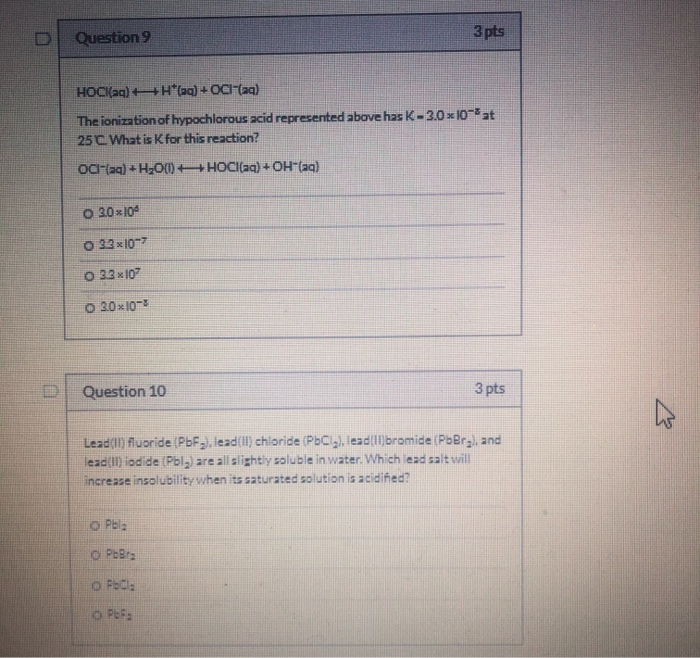Question 9 3 pts HOCl(aq) +H*(aq) + OCI (aq) The ionization of hypochlorous acid represented above has K-3.0x10-8 at 25 C. What is K for this reaction? OCI-(aq) + H200)+HOCl(aq) + OH-(aq) O 30x10 0 33x10-7 O 33x10 0 30x10-3 Question 10 3 pts Lead() fluoride (PbF), lead(II) chloride ( PC), lead()bromide (PbBrg, and lead(II) iodide (Pbly are all slightly soluble in water. Which lead salt will increase insolubility when its saturated solution is acidified? OPbla O PbBra O Pbda...

• ### Can you please try to answer these four questions for me? 3 pts Question 22 20.0mL...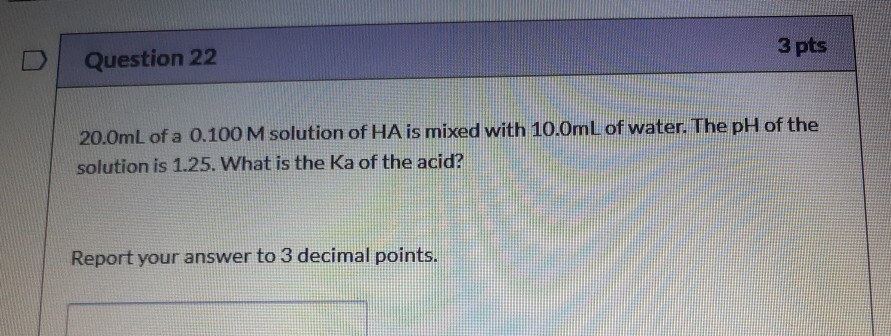Can you please try to answer these four questions for me? 3 pts Question 22 20.0mL of a 0.100 M solution of HA is mixed with 10.0mL of water. The pH of the solution is 1.25. What is the Ka of the acid? Report your answer to 3 decimal points. 3 p Question 27 Determine the molar solubility of an ionic compound with the general formula X,Y, where X is the cation and Y is the anion, given a Ksp...